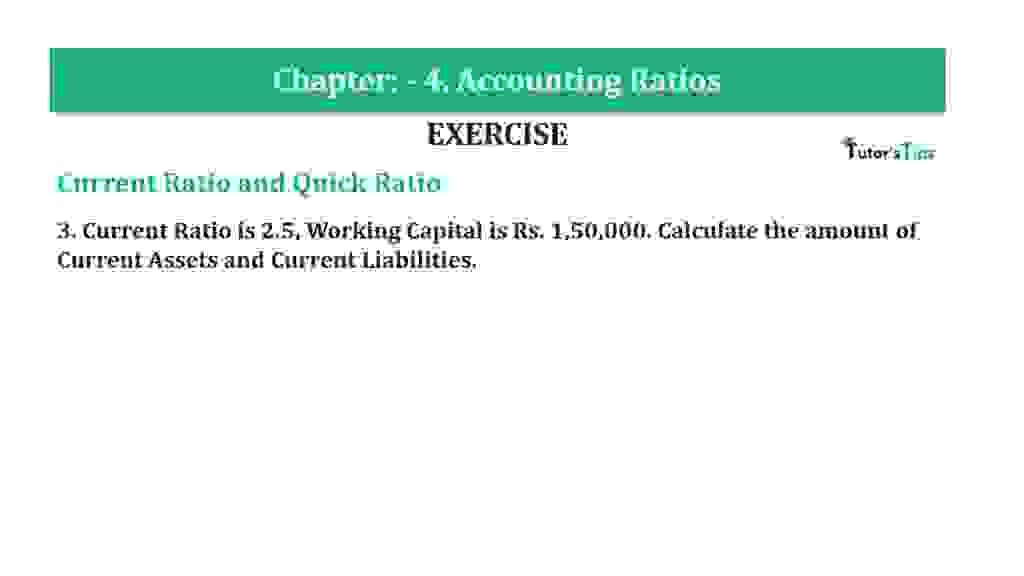# Question 3 Chapter 4 of +2-B – T.S. Grewal 12 ClassQuestion 3 Chapter 4 of +2-B

Current Ratio and Quick Ratio

3. Current Ratio is 2.5, Working Capital is Rs. 1,50,000. Calculate the number of Current Assets and Current Liabilities.

### The solution of Question 3 Chapter 4 of +2-B: –

 Current Ratio= Current Assets Current Liabilities
 Current Ratio= 2.5
 Current Liabilities= Trade Payables + Other Current Liabilities

 = Rs.90,000+ Rs.2,10,000 = Rs.3,00,000

 Working Capital= Current Assets – Current Liabilities

 Rs. 1,50,000= Current Assets – Current Liabilities

 Rs. 1,50,000 = 2.5 CL – CL

 1.5 Current Liabilities= Rs. 1,50,000

 Current Liabilities = Rs. 1,00,000

 Current Assets= 2.5 x Rs. 1,00,000
 Rs.2,50,000

Balance Sheet: Meaning, Format & Examples

Thanks, Please Like and share with your friends

Comment if you have any question.

Also, Check out the solved question of previous Chapters: –

### T.S. Grewal’s Double Entry Book Keeping (Vol. II: Accounting for Companies)T.S. Grewal’s Analysis of Financial Statements